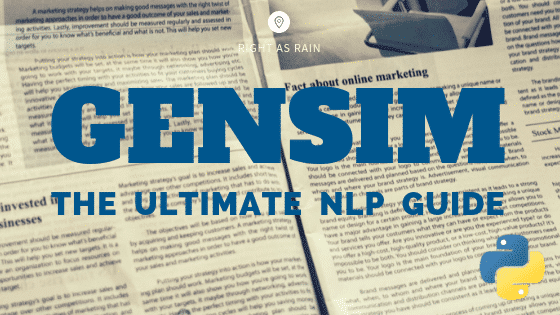# Soft Cosine Similarity## Cosine Similarity – Understanding the math and how it works (with python codes)

Cosine similarity is a metric used to measure how similar the documents are irrespective of their size. Mathematically, it measures the cosine of the angle between two vectors projected in a multi-dimensional space. The cosine similarity is advantageous because even if the two similar documents are far apart by the Euclidean distance (due to the …## Gensim Tutorial – A Complete Beginners Guide

Gensim is billed as a Natural Language Processing package that does ‘Topic Modeling for Humans’. But it is practically much more than that. It is a leading and a state-of-the-art package for processing texts, working with word vector models (such as Word2Vec, FastText etc) and for building topic models. Gensim Tutorial – A Complete Beginners …Course Preview

## Machine Learning A-Z™: Hands-On Python & R In Data Science

### Free Sample Videos:#### Machine Learning A-Z™: Hands-On Python & R In Data Science#### Machine Learning A-Z™: Hands-On Python & R In Data Science#### Machine Learning A-Z™: Hands-On Python & R In Data Science#### Machine Learning A-Z™: Hands-On Python & R In Data Science